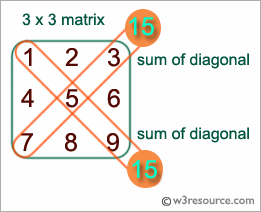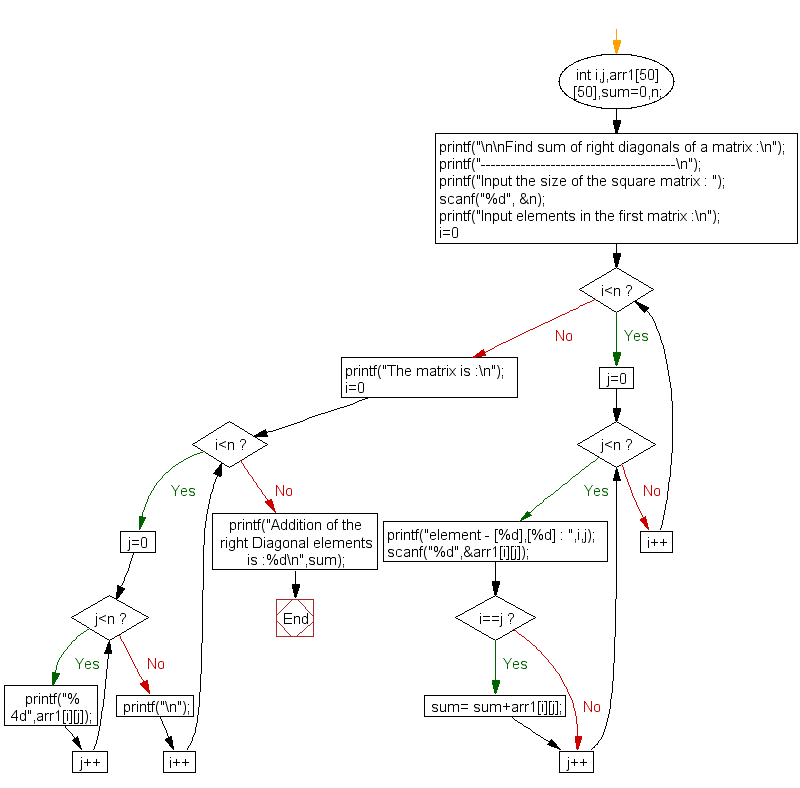﻿ C exercises: Find sum of right diagonals of a matrix - w3resource# C Exercises: Find sum of right diagonals of a matrix

## C Array: Exercise-23 with Solution

Write a program in C to find sum of right diagonals of a matrix.

Pictorial Presentation:Sample Solution:

C Code:

``````#include <stdio.h>

void main()

{
int i,j,arr1,sum=0,n;

printf("\n\nFind sum of right diagonals of a matrix :\n");
printf("---------------------------------------\n");

printf("Input the size of the square matrix : ");
scanf("%d", &n);
printf("Input elements in the first matrix :\n");
for(i=0;i<n;i++)
{
for(j=0;j<n;j++)
{
printf("element - [%d],[%d] : ",i,j);
scanf("%d",&arr1[i][j]);
if (i==j) sum= sum+arr1[i][j];
}
}

printf("The matrix is :\n");
for(i=0;i<n;i++)
{
for(j=0;j<n ;j++)
printf("% 4d",arr1[i][j]);
printf("\n");
}

printf("Addition of the right Diagonal elements is :%d\n",sum);
}
```
```

Sample Output:

```Find sum of right diagonals of a matrix :
---------------------------------------
Input the size of the square matrix : 2
Input elements in the first matrix :
element - , : 1
element - , : 2
element - , : 3
element - , : 4
The matrix is :
1   2
3   4
Addition of the right Diagonal elements is :5
```

Flowchart:C Programming Code Editor:

Improve this sample solution and post your code through Disqus.

What is the difficulty level of this exercise?

﻿

## C Programming: Tips of the Day

Where is the C auto keyword used?

auto is a modifier like static. It defines the storage class of a variable. However, since the default for local variables is auto, you don't normally need to manually specify it.

Ref : https://bit.ly/3yzwC9r